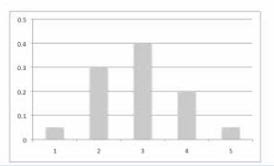## Special Offers on Trainings

This blog has a tie up with Many top online training providers who are offering great deals for readers of this blog. The certifications covered include PMP, PMI RMP, PMI ACP, CAPM, Scrum Master Certification etc.

Click here to check them out.

## Saturday, February 16, 2013

### Discrete Distributions

In the previous chapter, we learnt about the Continuous distributions. As a continuation, we are going to cover the other type of probability distributions which is “Discrete Distribution”

Remember that, just like the continuous distributions, the discrete distributions too are part of the Data Gathering and Representation Techniques sub-group of the tools and techniques in quantitative risk analysis.

Another point to note here is the fact that, the PMBOK Guide gives only a very high level or brief description of discrete distributions. We too will be covering only the basic facts (probably in a bit more detail when compared to the PMBOK but not in great detail) that are essential for you to know and understand from the PMI RMP Examination perspective.

Discrete Distributions are

• Used to show uncertain events where the probability of occurrence can be calculated accurately
• Based on a whole number

Discrete Distributions are used to represent:
• Possible scenarios in a decision tree
• Results of a prototype or
• Results of a test

The following is a sample discrete distribution:As you can see, it contains various bars, each of which represents a possible outcome/option. Each outcome is assigned a probability and the sum of all of the outcomes must add up to 1 or 100%

For ex: If you flip a coin, there is a 50% chance that you will get a head and another 50% for the tail. So, if we sum up the probability of both these outcomes you get 100%. If you create a Discrete Distribution for this case, you will see two equal sized bars each towering up to 0.5 respectively.

There are several types of discrete distributions, like:
a. Discrete Uniform
b. Binomial
c. Hypergeometric
d. Etc…

The point here is that, these are not part of the exam syllabus and hence are out of scope of our current discussion. From the PMI RMP Exam perspective, all you need to remember about the discrete distributions is:

• They show uncertain events such as outcome of decisions or tests
• They represent several possible outcomes
• Each outcome is assigned a probability and each bar in the image represents an outcome
• The sum of all these probabilities works out to 1 or 100%
• They are used in decision tree analysis.

Prev: Continuous Distributions

Next: Sensitivity Analysis

#### Post a Comment

© 2013 by www.getpmpcertified.blogspot.com. All rights reserved. No part of this blog or its contents may be reproduced or transmitted in any form or by any means, electronic, mechanical, photocopying, recording, or otherwise, without prior written permission of the Author.